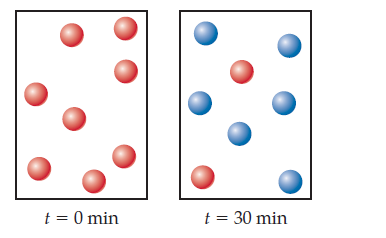×
Get Full Access to Chemistry: The Central Science - 12 Edition - Chapter 14 - Problem 8e
Get Full Access to Chemistry: The Central Science - 12 Edition - Chapter 14 - Problem 8e

×

Visualizing Concepts(a) Given the following diagrams at tISBN: 9780321696724 27

Solution for problem 8E Chapter 14

Chemistry: The Central Science | 12th Edition

• Textbook Solutions
• 2901 Step-by-step solutions solved by professors and subject experts
• Get 24/7 help from StudySoup virtual teaching assistantsChemistry: The Central Science | 12th Edition

4 5 1 342 Reviews
30
2
Problem 8E

(a) Given the following diagrams at t = 0 min and t = 30 min, what is the half-life of the reaction if it follows first-order kinetics?(b) After four half-life periods for a first-order reaction, what fraction of reactant remains? [Section 14.4]

Step-by-Step Solution:
Step 1 of 3

9/21 Lecture Notes A History of Life on Earth  HANDOUT → geological eras and periods *MUST MEMORIZE*  Points on Rocks  Sedimentary Rocks  Form mud, clay, sand, and gravel  Contains fossils  Cannot be dated or aged directly  Age is determined by fossils  Igneous Rocks  Form from lava, magma → melted rocks  Contain no fossils  Can be aged with radioactive elements  How to get an absolute age of rocks  Use radioactive decay  Radioactive decay rates are constant  Key elements  C → N Age range: 100 – 50,000 years ago  Information is in 14C/12C ratio  High ratio: young  Low ratio: old  K → Ar Age range: 10 million – 4.5 b

Step 2 of 3

Step 3 of 3

ISBN: 9780321696724

Since the solution to 8E from 14 chapter was answered, more than 542 students have viewed the full step-by-step answer. The full step-by-step solution to problem: 8E from chapter: 14 was answered by , our top Chemistry solution expert on 04/03/17, 07:58AM. This full solution covers the following key subjects: min, reaction, order, life, half. This expansive textbook survival guide covers 49 chapters, and 5471 solutions. This textbook survival guide was created for the textbook: Chemistry: The Central Science, edition: 12. The answer to “?(a) Given the following diagrams at t = 0 min and t = 30 min, what is the half-life of the reaction if it follows first-order kinetics? (b) After four half-life periods for a first-order reaction, what fraction of reactant remains? [Section 14.4]” is broken down into a number of easy to follow steps, and 43 words. Chemistry: The Central Science was written by and is associated to the ISBN: 9780321696724.

Unlock Textbook Solution